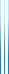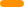# PublicationsPapers

## Approximate polymorphisms

Gilad Chase, Yuval Filmus, Dor Minzer, Elchanan Mossel, and Nitin Saurabh
Submitted

A function $f\colon \{0,1\}^n \to \{0,1\}$ is a polymorphism of $g\colon \{0,1\}^m \to \{0,1\}$ if $f \circ g^n = g \circ f^m$. For example, $f$ is a polymorphism of $g(x,y) = x \oplus y$ if $f(x \oplus y) = f(x) \oplus f(y)$. Dokow and Holzman determined all polymorphisms of $g$ for arbitrary $g$.

What happens if $f$ only satisfies $f \circ g^n = g \circ f^m$ for most inputs? Extending earlier work on the case $g = \mathsf{AND}$, we show that in most cases, $f$ must be close to an exact polymorphism. When $g = \mathsf{NAND}$ or $g = \mathsf{NOR}$, we show that $f$ must be close to $\mathsf{AND}$ or $\mathsf{OR}$ (respectively).

We also discuss the list-decoding regime, showing that for each $g \neq \mathsf{XOR},\mathsf{NXOR}$ there is a constant $s_g < 1$ such that if $f \circ g^n = g \circ f^m$ holds with probability above $s_g$, then $f$ correlates with some low-degree character. When $g = \mathsf{AND}$, we determine the optimal value of $s_g$ to be roughly 0.815.

## BibTeX

@misc{CFMMS2021,
author = {Gilad Chase and Yuval Filmus and Dor Minzer and Elchanan Mossel and Nitin Saurabh},
title = {Approximate polymorphisms},
year = {2021}
}copy to clipboard